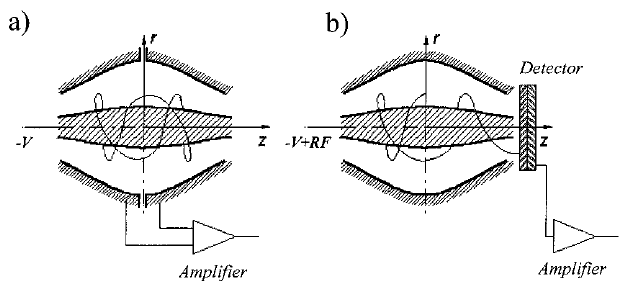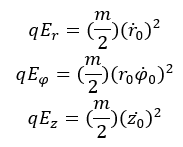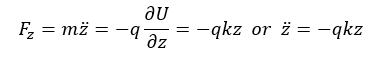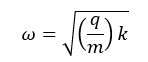$$\require{cancel}$$

# Mass Analyzer Orbitrap

Mass spectrometry (MS) is an analytical instrument that is used to measure the mass-to-charge ratios (m/z) of samples to obtain qualitative and quantitative information. The development of MS started around 1920 where it was used to study the isotopic abundance of elements. MS capabilities have increased greatly since 1920. Within the last 90 years, MS has been used to study complex organic and inorganic mixtures, the composition of the solid surface, biological samples and much more[1-2]. Today, MS comes in many forms because of its tandem capabilities with other analytical instruments[1-2]. This allows MS to be very versatile making it one of most used analytical instruments today. One of the newest advancements in MS is the Orbitrap mass analyzer and that allows researchers to characterize biological samples such as cell membrane.

# Fundamentals

The principle of MS is based on separation of m/z ratios and analyzing them to gain a mass spectrum of the sample[1-2]. MS has three major components which are the ionization source, mass analyzer, and detector[1-2]. The overall schematic of an MS instrument is shown in Figure 1.Figure 1: Basic box diagram of a Mass Spectrometer 

Samples are injected using an inlet system that purifies the analyte, and subsequently becomes ionized. The standard method of ionization for Orbitrap mass analyzer is using Electrospray Ionization (ESI) . After ionization, the mass analyzer filters the samples for desired m/z ratios. In this case, the Orbitrap is the mass analyzer of interest. The desired m/z ions are then flown through to the detector to be processed into a mass spectrum. This signal is processed which gives us the readout. Creating an ion-free path is important to guide ions to the detector because vacuums (10-5 to 10-8) torr minimize the chance that ions will inadvertently collide with other molecules. If this unintentional collision occurs, a diminished signal may result By using a high vacuum system, the ion can transition through the MS with a low probability of collision with other ions.

# Ionization

Ionization methods can be classified as either soft or hard ionization[1-2]. Hard ionization methods create ions with high residual energy[1-2]. This method usually results in fragmentation of the molecule[1-2]. For hard ionization, electron ionization is one of the most common methods used in MS to produce ions for MS analysis. Unlike hard ionization, soft ionization methods use a lower residual energy to ionize samples where fragmentation is less likely to occur. This is ideal when studying biological samples where sample integrity is important[1-2]. ESI is an example of soft ionization method for MS[1-2]. For Orbitrap mass analyzer, ESI is the most common method used for the ionization source [1-2]. BUsing the mass analyzer, charged ions can be created and their movements controlled by magnetic and electric fields.

# Mass Analyzer

The mass analyzer is one of the most vital components of the MS. It filters the ion for the desired m/z ratios to allow the detector to output the mass spectrum[1-2]. Beside filtering m/z ratio, they also can act as an ion storage center, fragmentation chamber or even an ion ejection compartment[1-2]. In the Orbitrap mass analyzer, both a quadrupole and Orbitrap are present[1-2]. The quadrupole component allows for mass selection and guides ions with the desired m/z to the Orbitrap[1-2].

The Orbitrap is an ion trap-based mass analyzer developed from the Kingdon trap[2-6]. Developed in 1923 by K.H. Kingdon, the Kingdon trap was originally created to explore electric potential in an infinitely long electrode. Figure 2 below shows the schematic diagram of the Kingdon trap.Figure 2: Kingdon trap schematic diagram 

60 years after K.H. Kingdon passed, R.D. Knight, one of K.H. Kingdon’s contemporaries developed the Knight trap based on K.H. Kingdon’s original work. The Knight trap improved upon the Kingdon trap’s limitations by deriving m/z from electrical potentials . Even though R.D. Knight was able to create the connection between the electric potential of an electrode to m/z ratio, the Knight trap still had its limitation. The Knight trap had issues in discriminating between similar m/z ratios and the focus of R.D. Knight work was on ion ejection from the Knight trap.

In the year 2000, a Russian physicist Alexander Alexeyevich Makarov published his work on the orbitrap that resolved the limitation of the Knight trap. During the next five years, the Orbitrap mass analyzer became commercially available, allowing researchers to measure m/z ratios of large biological samples. Figure 3 shows the original design of the Orbitrap invented by Dr. Makarov. The Orbitrap gained great interest for MS analysis of biological samples because of its high resolution and high mass limit. Most other mass analyzers are limited to molecules 2 kDa/z to 4 kDa/ in size[2,6]. This would require having samples to have a charge greater than 50 to be able to measure that sample with the mass of 100kDa. In the case of the Orbitrap, the resolution limits are from 50kDa/z to 100kDa/z. For a biological samples weighing 100kDa, we would only need a charge of 1 to detect it in the Orbitrap. This is due to the high sensitivity of the Orbitrap having one of the highest resolution limits compared to other mass analyzers.Figure 3: Alexeyevich Makarov Orbitrap design 

# Orbitrap Theory

The Orbitrap mass analyzer operates by oscillating ions in a cylindrical electrode with tapered ends and detects signals for m/z based on Fourier transforms (FT)[2-3,6]. The electrode is powered by an RF frequency that makes the ion oscillate around the inner electrode. A better way to picture the details of an Orbitrap is shown in Figure 4 below shows a side view and cross-sections of the Orbitrap as ions circulate through the trap (in red)3].Figure 4: Orbitrap cross section and side view of an ion trajectory 

In the initial inject of the ions in the Orbitrap will results in Figure 4 part a where there is thin line demonstrating the trajectory. Try “When ions are initially injected, ions typically follow an imperfect oscillatory motion, with frequencies out-of-phase with the electrode (Figure 4 a, grey lines). As time progresses, hundreds of oscillations become incorporated into the Orbitrap and follow more in-phase circular revolutions (Figure 4 b, black region). However, if the initial injection of ions resonates with the electrode, a circular orbit can be obtained from the beginning (Figure 4 c, grey circle). The electrostatic potential of the Orbitrap can be explained with the equation:(1.1) [2,6]

The potential inside of the trap is based on the r and z cylindrical coordinates, field curvature k, the characteristic radius Rm (radius at when the ions are repelling instead of attracting), and a constant value C [2,6] . From Equation 1.1, one can deduce the shape of the axial along the z-axis that is represented as:(1.2) 

When z is set to zero, one can see that the maximum radii of the electrodes are that of R1,2.

Going back to the ion trajectory, there must be some initial conditions that are needed to be met when at t=0 These conditions are listed as:(1.3) 

With these following conditions, then one can get three equations that represent the polar coordinates system of the Orbitrap listed as:(1.4) 

These three polar coordinates equation each has their own energy equation associated with them shown as:(1.5) 

By meeting the condition set in Equation 1. 4, one can determine the ion initial kinetic energy which will be the sum of all three energy in Equation 1.5. The more important thing is that Ez is the energy factor of the harmonic oscillator for the Orbitrap. Going back to Equation 1.1, one can find the voltage gradient needed for any given system shown as:(1.6) 

The voltage gradient is focused only along the z-axis of the Orbitrap. This voltage gradient can be further explained by the equation below:(1.7) 

The force induces by the electric field on the z-axis is described in Equation 1.7 which the exact solution is given below as:(1.8) 

Equation 1.8 correlate frequency measure in the Orbitrap is only dependant on the z-axis shown below:(1.9) 

From this final equation, one can see the relation of the frequency with mass-to-charge ratio while being independent of kinetic energy. This is how FT plays an important role in getting the mass spectrum when using an Orbitrap mass analyzer. Shown in Equation 1.9 we can see a relationship between the m/z to frequency. With the Orbitrap mass analyzer, one can surpass the limits of biomolecule sample for MS analysis which are high masses biological samples.The mass limit resolution range for quadrupole is 2,000 m/z to 4000 m/z while the mass limit resolution range for Orbitrap is 50,000 m/z to 100,000 m/z. This is up to two magnitude difference in mass by using an Orbitrap mass analyzer.

# Detector

Unlike other MS instruments, the detector of an Orbitrap mass analyzer is built into the Orbitrap itself[3,6]. "Figure 5"Figure 5: A side view schematic of the Orbitrap mass analyzer

The detector for the Orbitrap is an image current detector. For the Orbitrap, an electrostatic field is produced in the mass analyzer by the ion that are being measured through a series of signal processing. The signal measured can be explained below:2.1

Equation 2.1 uses Green’s reciprocity theorem to get the signal voltage induced by the oscillating ion in the Orbitrap This equation shows the relationship between image charge (Q) induce the electrode to the charge (q) at point (r,φ,z) coordinate. The voltage obtained in Equation 2.1, can be used to derive the induced current of the Orbitrap, as shown in Equation 2.2 below:.2.2

Equation 2.2 is the total image current for a cloud of ions in the Orbitrap stating the total image current is based on the summation of individual currents. An average value of r and z would be used for this system. When talking about a One ion-based system, the z-axis will be the main thing that needs to determine the m/z ratio but when multiple ions are added to the system, one would need the radial axis to differentiate between the different ions. These are dependent on the different energy associated with each polar coordinate shown in Equation 1.5. The total image will be determined by the equation below:2.3

Equation 2.3 gives the total image current where it can describe the effective gap (lambda) between the detection electrodes. With these equations and using FT, one can get a spectrum that is shown in Figure 6 and get mass spectrum data in Figure 7 . Figure 6 is 56Fe+ element that oscillated thousands of times around the Orbitrap mass analyzer and Figure 7 is a FT of the data giving the mass spectrum.Figure 6: Signal of a 56Fe+ ion in the OrbitrapFigure 7: FT of theSignal of a 56Fe+ ion in the Orbitrap

# Applications

One of the major application of Orbitrap mass analyzer is to use it to study large biomolecule such as cell membrane. Some of the early work using Orbitrap mass analyzer was to study bovine insulin which was done before in the past but unable to get clear MS peaks[2-3]. Figure 8 gives the mass spectrum data of bovine insulin that illustrated good resolution peaks that was not obtainable without the orbitrap. This shows the capability of Orbitrap mass analyzer where one get a resolution of 100,000 m/zFigure 8 The MS data of Bovine Insulin using ESI Orbitrap mass analyzer 

These days Orbitrap mass analyzer have been used in protein analysis such as proteomic, glycoproteomic, and oligopeptide[7-8]. These can be applied to cell membrane analysis [7-8].The reason why Orbitrap is ideal because it is able to differentiate between complex biological samples that have similar m/z ratio. Orbitrap is not the only MS that can be used for cell membrane analysis. Figure 9 gives all the possible ionizer and mass analyzer that could be coupled with one another to conduct MS analysis but base on resolution capabillity, Orbitrap mass analyzer is the best.Figure 9: Different components that can be used for MS analysis of cell membranes

The advent of using Orbitrap over other MS instrument due to the high-resolution capability of the Orbitrap[2,9]. Due to the high sensitivity of the Orbitrap, one can measure multiple concomitant bindings event that were not possible before. The high sensitivity allows the Orbitrap to distinguish small mass changes. One biological application are lipids, where their mass difference can be small. Using the Orbitrap, one can distinguish mass difference as small as 12Da which were not possible for older generation MS. Beside being able to distinguish low mass molecule, Orbitrap can get good spectra of high mass biological molecules.Another advantage of the Orbitrap is to study proteoforms, glycoforms and post-translational modifications that occur on the cell membranes. A recent paper was published Nature Methods discuss current Orbitrap applications on membrane protein and lipid binding. This paper can be link to by this doi: 10.1038/nmeth.3771 

# Summary

Orbitrap mass analyzer has opened a new path for biologically analysis, since it has high resolution limit of 100kDa/z. This makes Orbitrap a power tool in understand large biological system that could normally not be study with older generation MS. Orbitrap are currently being applied into the field proteomic, glycoprotemic, and glycolips which can be cell membrane related[7-9]. Even though Orbitrap mass analyzer has advanced our studies in the biological system, there at still limitation to the instrument[2-3,7-9]. With that said, Orbitrap still has a lot of potential for future advancement that can surpass its current limitation which helps the researcher in the field of biophysic.

# References

1. Skoog, D. A.; Leary, J. J., Principles of Instrumental Analysis. Saunders College Publishing: Philadelphia, PA, 1992; p 420-461.
2. Hoffmann, E. D.; Stroobant, V., Mass spectrometry: principles and applications. John Wiley & Sons Ltd: Chicester, West Sussex, 2007; p 1-503.
3. Hu, Q.; Noll, R. J.; Li, H.; Makarov, A.; Hardman, M.; Graham Cooks, R., The Orbitrap: a new mass spectrometer. J Mass Spectrom 2005, 40 (4), 430-43.
4. Kingdon, K. H., A Method for the Neutralization of Electron Space Charge by Positive Ionization at Very Low Gas Pressures. Physical Review 1923, 21 (4), 408-418.
5. Knight, R. D., Storage of ions from laser‐produced plasmas. Applied Physics Letters 1981, 38 (4), 221-223.
6. Makarov, A., Electrostatic Axially Harmonic Orbital Trapping: A High-Performance Technique of Mass Analysis. Analytical Chemistry 2000, 72 (6), 1156-1162.
7. Perry, R. H.; Cooks, R. G.; Noll, R. J., Orbitrap mass spectrometry: instrumentation, ion motion and applications. Mass Spectrom Rev 2008, 27 (6), 661-99.
8. Kailemia, M. J.; Xu, G.; Wong, M. Y.; Li, Q.; Goonatilleke, E.; Leon, F.; Lebrilla, C. B., Recent Advances in the Mass Spectrometry Methods for Glycomics and Cancer. Analytical Chemistry 2017.
9. Calabrese, A. N.; Radford, S. E., Mass spectrometry-enabled structural biology of membrane proteins. Methods 2018.
10. Gault, J.; Donlan, J. A. C.; Liko, I.; Hopper, J. T. S.; Gupta, K.; Housden, N. G.; Struwe, W. B.; Marty, M. T.; Mize, T.; Bechara, C.; Zhu, Y.; Wu, B.; Kleanthous, C.; Belov, M.; Damoc, E.; Makarov, A.; Robinson, C. V., High-resolution mass spectrometry of small molecules bound to membrane proteins. Nature methods 2016, 13 (4), 333- 336.